# 3148 Results

View
Selected filters:
• Mathematics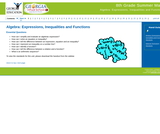Conditional Remix & Share Permitted
CC BY-NC-SA
Rating

Middle Grades Math 8 Algebra Part One

Subject:
Mathematics
Material Type:
Module
Provider:
Georgia Virtual
Author:
Georgia Virtual School
06/02/2018Conditional Remix & Share Permitted
CC BY-NC-SA
Rating

Students will explore multi-digit numbers and the relationship between ones, tens and hundreds; a digit in one place is 10x the digit in the place to its right. Students will use their bodies to represent digits in multi-digit numbers up to the hundredths place and compare these numbers using <, =, >. Students will use their bodies as multi-digit numbers to add and subtract.

Subject:
Mathematics
Material Type:
Activity/Lab
Teaching/Learning Strategy
Author:
02/08/2019Educational Use
Rating

In this video segment from Cyberchase, the CyberSquad replaces a piece of track to get the Madre Bonita Express to the Mother's Day harvest.

Subject:
Mathematics
Material Type:
Lecture
Provider:
PBS LearningMedia
Author:
U.S. Department of Education
WNET
07/24/2008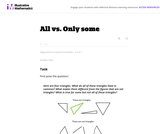Unrestricted Use
CC BY
Rating

This is a task from the Illustrative Mathematics website that is one part of a complete illustration of the standard to which it is aligned. Each task has at least one solution and some commentary that addresses important asects of the task and its potential use. Here are the first few lines of the commentary for this task: First pose the question: Here are four triangles. What do all of these triangles have in common? What makes them different from the figures that are no...

Subject:
Mathematics
Material Type:
Activity/Lab
Provider:
Illustrative Mathematics
Provider Set:
Illustrative Mathematics
Author:
Illustrative Mathematics
05/24/2013Unrestricted Use
CC BY
Rating

This is a task from the Illustrative Mathematics website that is one part of a complete illustration of the standard to which it is aligned. Each task has at least one solution and some commentary that addresses important asects of the task and its potential use. Here are the first few lines of the commentary for this task: Materials * A copy of Grandfather Tang's Story by Ann Tompert * One set of tangrams for each student (see note in commentary) * A set of tangrams for t...

Subject:
Mathematics
Material Type:
Activity/Lab
Provider:
Illustrative Mathematics
Provider Set:
Illustrative Mathematics
Author:
Illustrative Mathematics
05/29/2013Unrestricted Use
CC BY
Rating

This is a task from the Illustrative Mathematics website that is one part of a complete illustration of the standard to which it is aligned. Each task has at least one solution and some commentary that addresses important asects of the task and its potential use. Here are the first few lines of the commentary for this task: Materials - 2 clear plastic cups for each pair of students - 4 bean seeds for each pair - soil - unifix cubes - a plant or math journal to record data ...

Subject:
Mathematics
Material Type:
Activity/Lab
Provider:
Illustrative Mathematics
Provider Set:
Illustrative Mathematics
Author:
Illustrative Mathematics
04/17/2013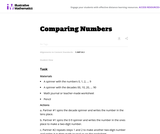Unrestricted Use
CC BY
Rating

This is a task from the Illustrative Mathematics website that is one part of a complete illustration of the standard to which it is aligned. Each task has at least one solution and some commentary that addresses important asects of the task and its potential use. Here are the first few lines of the commentary for this task: Materials A spinner with the numbers 0, 1, 2, ... 9 A spinner with the decades 00, 10, 20, ... 90 Math journal or teacher-made worksheet Pencil Actions...

Subject:
Mathematics
Material Type:
Activity/Lab
Provider:
Illustrative Mathematics
Provider Set:
Illustrative Mathematics
Author:
Illustrative Mathematics
04/19/2013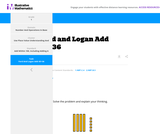Unrestricted Use
CC BY
Rating

This is a task from the Illustrative Mathematics website that is one part of a complete illustration of the standard to which it is aligned. Each task has at least one solution and some commentary that addresses important aspects of the task and its potential use.

Subject:
Mathematics
Material Type:
Activity/Lab
Provider:
Illustrative Mathematics
Provider Set:
Illustrative Mathematics
Author:
Illustrative Mathematics
11/17/2020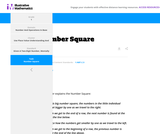Unrestricted Use
CC BY
Rating

This is a task from the Illustrative Mathematics website that is one part of a complete illustration of the standard to which it is aligned. Each task has at least one solution and some commentary that addresses important aspects of the task and its potential use.

Subject:
Mathematics
Material Type:
Activity/Lab
Provider:
Illustrative Mathematics
Provider Set:
Illustrative Mathematics
Author:
Illustrative Mathematics
11/17/2020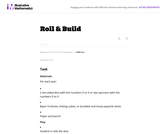Unrestricted Use
CC BY
Rating

This is a task from the Illustrative Mathematics website that is one part of a complete illustration of the standard to which it is aligned. Each task has at least one solution and some commentary that addresses important asects of the task and its potential use. Here are the first few lines of the commentary for this task: Materials For each pair: * 2 ten-sided dice with the numbers 0 to 9 or two spinners with the numbers 0 to 9 * Base-10 blocks, linking cubes, or bundled...

Subject:
Mathematics
Material Type:
Activity/Lab
Provider:
Illustrative Mathematics
Provider Set:
Illustrative Mathematics
Author:
Illustrative Mathematics
05/24/2013Unrestricted Use
CC BY
Rating

This is a task from the Illustrative Mathematics website that is one part of a complete illustration of the standard to which it is aligned. Each task has at least one solution and some commentary that addresses important asects of the task and its potential use. Here are the first few lines of the commentary for this task: Materials Set of cards from 1-100, these can be purchased or created using a black marker and index cards Setup Sort the 1-100 cards into sequential gr...

Subject:
Mathematics
Material Type:
Activity/Lab
Provider:
Illustrative Mathematics
Provider Set:
Illustrative Mathematics
Author:
Illustrative Mathematics
03/16/2013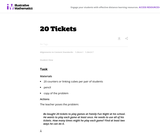Unrestricted Use
CC BY
Rating

This is a task from the Illustrative Mathematics website that is one part of a complete illustration of the standard to which it is aligned. Each task has at least one solution and some commentary that addresses important asects of the task and its potential use. Here are the first few lines of the commentary for this task: Materials 20 counters or linking cubes per pair of students pencil copy of the problem Actions The teacher poses the problem: Bo bought 20 tickets to p...

Subject:
Mathematics
Material Type:
Activity/Lab
Provider:
Illustrative Mathematics
Provider Set:
Illustrative Mathematics
Author:
Illustrative Mathematics
05/05/2013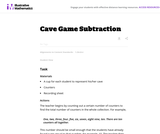Unrestricted Use
CC BY
Rating

This is a task from the Illustrative Mathematics website that is one part of a complete illustration of the standard to which it is aligned. Each task has at least one solution and some commentary that addresses important asects of the task and its potential use. Here are the first few lines of the commentary for this task: Materials * A cup for each student to represent his/her cave * Counters * Recording sheet Actions The teacher begins by counting out a certain number o...

Subject:
Mathematics
Material Type:
Activity/Lab
Provider:
Illustrative Mathematics
Provider Set:
Illustrative Mathematics
Author:
Illustrative Mathematics
03/25/2014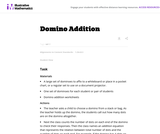Unrestricted Use
CC BY
Rating

This is a task from the Illustrative Mathematics website that is one part of a complete illustration of the standard to which it is aligned. Each task has at least one solution and some commentary that addresses important asects of the task and its potential use. Here are the first few lines of the commentary for this task: Materials A large set of dominoes to affix to a whiteboard or place in a pocket chart, or a regular set to use on a document projector. One set of domi...

Subject:
Mathematics
Material Type:
Activity/Lab
Provider:
Illustrative Mathematics
Provider Set:
Illustrative Mathematics
Author:
Illustrative Mathematics
05/24/2013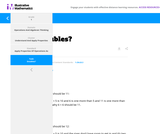Unrestricted Use
CC BY
Rating

This is a task from the Illustrative Mathematics website that is one part of a complete illustration of the standard to which it is aligned. Each task has at least one solution and some commentary that addresses important aspects of the task and its potential use.

Subject:
Algebra
Material Type:
Activity/Lab
Provider:
Illustrative Mathematics
Provider Set:
Illustrative Mathematics
Author:
Illustrative Mathematics
11/17/2020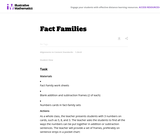Unrestricted Use
CC BY
Rating

This is a task from the Illustrative Mathematics website that is one part of a complete illustration of the standard to which it is aligned. Each task has at least one solution and some commentary that addresses important asects of the task and its potential use. Here are the first few lines of the commentary for this task: Materials * Fact Family work sheets * Blank addition and subtraction frames (2 of each) * Numbers cards in fact-family sets Actions As a whole class, t...

Subject:
Mathematics
Material Type:
Activity/Lab
Provider:
Illustrative Mathematics
Provider Set:
Illustrative Mathematics
Author:
Illustrative Mathematics
09/06/2013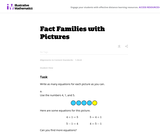Unrestricted Use
CC BY
Rating

This is a task from the Illustrative Mathematics website that is one part of a complete illustration of the standard to which it is aligned. Each task has at least one solution and some commentary that addresses important asects of the task and its potential use. Here are the first few lines of the commentary for this task: Write as many equations for each picture as you can. Use the numbers 4, 1, and 5. Here are some equations for this picture. 4+1=5 \hskip4em 5 = 4+1 5-1...

Subject:
Mathematics
Material Type:
Activity/Lab
Provider:
Illustrative Mathematics
Provider Set:
Illustrative Mathematics
Author:
Illustrative Mathematics
03/25/2014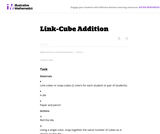Unrestricted Use
CC BY
Rating

This is a task from the Illustrative Mathematics website that is one part of a complete illustration of the standard to which it is aligned. Each task has at least one solution and some commentary that addresses important asects of the task and its potential use. Here are the first few lines of the commentary for this task: Materials * Link-cubes or snap-cubes (2 colors for each student or pair of students) * A die * Paper and pencil Actions 1. Roll the die. 2. Using a sin...

Subject:
Mathematics
Material Type:
Activity/Lab
Provider:
Illustrative Mathematics
Provider Set:
Illustrative Mathematics
Author:
Illustrative Mathematics
09/18/2013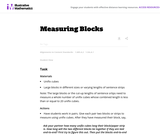Unrestricted Use
CC BY
Rating

This is a task from the Illustrative Mathematics website that is one part of a complete illustration of the standard to which it is aligned. Each task has at least one solution and some commentary that addresses important asects of the task and its potential use. Here are the first few lines of the commentary for this task: Materials * Unifix cubes * Large blocks in different sizes or varying lengths of sentence strips Note: The large blocks or the cut-up lengths of senten...

Subject:
Mathematics
Material Type:
Activity/Lab
Provider:
Illustrative Mathematics
Provider Set:
Illustrative Mathematics
Author:
Illustrative Mathematics
03/18/2014Unrestricted Use
CC BY
Rating

This is a task from the Illustrative Mathematics website that is one part of a complete illustration of the standard to which it is aligned. Each task has at least one solution and some commentary that addresses important asects of the task and its potential use. Here are the first few lines of the commentary for this task: Materials The Very Hungry Caterpillar by Eric Carle The students work individually or in pairs. Each student or pair needs: Three ten-frames for each s...

Subject:
Mathematics
Material Type:
Activity/Lab
Provider:
Illustrative Mathematics
Provider Set:
Illustrative Mathematics
Author:
Illustrative Mathematics
04/24/2013Educational Use
Rating

In this lesson children will be asked to make a graph, then listen to a story. After the story, they will do a similar graph and then compare the two graphs.

Subject:
Mathematics
Material Type:
Lesson Plan
Provider:
Utah Education Network
02/16/2021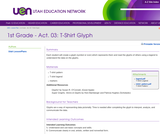Educational Use
Rating

In this lesson each student will create a glyph (symbol or icon) which represents them and read the glyphs of others using a legend to understand the data on the glyphs.

Subject:
Mathematics
Material Type:
Lesson Plan
Provider:
Utah Education Network
02/16/2021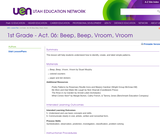Educational Use
Rating

This lesson will help students understand how to identify, create, and label simple patterns.

Subject:
Mathematics
Material Type:
Lesson Plan
Provider:
Utah Education Network
02/16/2021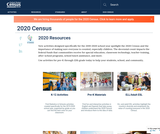Unrestricted Use
Public Domain
Rating

New activities designed specifically for the 2019-2020 school year spotlight the 2020 Census and the importance of making sure everyone is counted, especially children. The decennial count impacts the federal funds that communities receive for special education, classroom technology, teacher training, after-school programs, school lunch assistance, and more. PreK-grade 12.

Subject:
Statistics and Probability
Government
Material Type:
Activity/Lab
Author:
Us Census Bureau
11/06/2019Unrestricted Use
CC BY
Rating

This problem helps students practice adding three numbers whose sum are 20 or less.

Subject:
Mathematics
Material Type:
Activity/Lab
Provider:
Illustrative Mathematics
Provider Set:
Illustrative Mathematics
Author:
Illustrative Mathematics
09/10/2012Conditional Remix & Share Permitted
CC BY-NC-SA
Rating

This short video and interactive assessment activity is designed to give fifth graders an overview of 24-hour clocks.

Subject:
Mathematics
Material Type:
Assessment
Interactive
Lecture
Provider:
CK-12 Foundation
Provider Set:
CK-12 Elementary Math
11/18/2020Conditional Remix & Share Permitted
CC BY-NC-SA
Rating

This short video and interactive assessment activity is designed to give fourth graders an overview of 24-hour clocks.

Subject:
Mathematics
Material Type:
Assessment
Interactive
Lecture
Provider:
CK-12 Foundation
Provider Set:
CK-12 Elementary Math
11/18/2020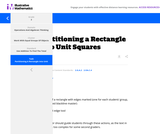Unrestricted Use
CC BY
Rating

This is a task from the Illustrative Mathematics website that is one part of a complete illustration of the standard to which it is aligned. Each task has at least one solution and some commentary that addresses important aspects of the task and its potential use.

Subject:
Geometry
Material Type:
Activity/Lab
Provider:
Illustrative Mathematics
Provider Set:
Illustrative Mathematics
Author:
Illustrative Mathematics
11/17/2020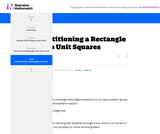Unrestricted Use
CC BY
Rating

This is a task from the Illustrative Mathematics website that is one part of a complete illustration of the standard to which it is aligned. Each task has at least one solution and some commentary that addresses important aspects of the task and its potential use.

Subject:
Geometry
Material Type:
Activity/Lab
Provider:
Illustrative Mathematics
Provider Set:
Illustrative Mathematics
Author:
Illustrative Mathematics
11/17/2020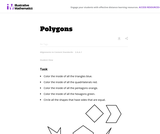Unrestricted Use
CC BY
Rating

This is a task from the Illustrative Mathematics website that is one part of a complete illustration of the standard to which it is aligned. Each task has at least one solution and some commentary that addresses important asects of the task and its potential use. Here are the first few lines of the commentary for this task: * Color the inside of all the triangles blue. * Color the inside of all the quadrilaterals red. * Color the inside of all the pentagons orange. * Color...

Subject:
Mathematics
Material Type:
Activity/Lab
Provider:
Illustrative Mathematics
Provider Set:
Illustrative Mathematics
Author:
Illustrative Mathematics
05/23/2013Unrestricted Use
CC BY
Rating

This is a task from the Illustrative Mathematics website that is one part of a complete illustration of the standard to which it is aligned. Each task has at least one solution and some commentary that addresses important asects of the task and its potential use. Here are the first few lines of the commentary for this task: Materials * Alexander, Who Used to be Rich Last Sunday by Judith Viorst * Plastic coins * Labels for items Alexander spent his money on (attached) * Pa...

Subject:
Mathematics
Material Type:
Activity/Lab
Provider:
Illustrative Mathematics
Provider Set:
Illustrative Mathematics
Author:
Illustrative Mathematics
06/23/2013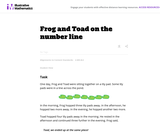Unrestricted Use
CC BY
Rating

This is a task from the Illustrative Mathematics website that is one part of a complete illustration of the standard to which it is aligned. Each task has at least one solution and some commentary that addresses important asects of the task and its potential use. Here are the first few lines of the commentary for this task: One day, Frog and Toad were sitting together on a lily pad. Some lily pads were in a line across the pond. In the morning, Frog hopped three lily pads ...

Subject:
Mathematics
Material Type:
Activity/Lab
Provider:
Illustrative Mathematics
Provider Set:
Illustrative Mathematics
Author:
Illustrative Mathematics
03/16/2013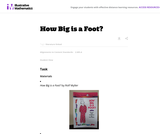Unrestricted Use
CC BY
Rating

This is a task from the Illustrative Mathematics website that is one part of a complete illustration of the standard to which it is aligned. Each task has at least one solution and some commentary that addresses important asects of the task and its potential use. Here are the first few lines of the commentary for this task: Materials * How Big is a Foot? by Rolf Myller * Masking tape * Enough 12-inch (one foot) rulers for every student or pair of students * One yardstick *...

Subject:
Mathematics
Material Type:
Activity/Lab
Provider:
Illustrative Mathematics
Provider Set:
Illustrative Mathematics
Author:
Illustrative Mathematics
05/22/2013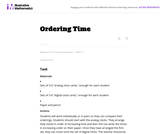Unrestricted Use
CC BY
Rating

This is a task from the Illustrative Mathematics website that is one part of a complete illustration of the standard to which it is aligned. Each task has at least one solution and some commentary that addresses important asects of the task and its potential use. Here are the first few lines of the commentary for this task: Materials * Sets of 3-6 "analog clock cards," enough for each student * Sets of 3-6 "digital clock cards," enough for each student * Paper and pencil A...

Subject:
Mathematics
Material Type:
Activity/Lab
Provider:
Illustrative Mathematics
Provider Set:
Illustrative Mathematics
Author:
Illustrative Mathematics
05/22/2013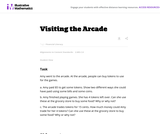Unrestricted Use
CC BY
Rating

This is a task from the Illustrative Mathematics website that is one part of a complete illustration of the standard to which it is aligned. Each task has at least one solution and some commentary that addresses important asects of the task and its potential use. Here are the first few lines of the commentary for this task: Amy went to the arcade. At the arcade, people can buy tokens to use for the games. Amy paid \\$5 to get some tokens. Show two different ways she could h...

Subject:
Mathematics
Material Type:
Activity/Lab
Provider:
Illustrative Mathematics
Provider Set:
Illustrative Mathematics
Author:
Illustrative Mathematics
03/24/2013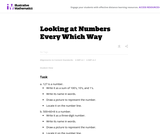Unrestricted Use
CC BY
Rating

This is a task from the Illustrative Mathematics website that is one part of a complete illustration of the standard to which it is aligned. Each task has at least one solution and some commentary that addresses important asects of the task and its potential use. Here are the first few lines of the commentary for this task: 127 is a number. Write it as a sum of 100's, 10's, and 1's. Write its name in words. Draw a picture to represent the number. Locate it on the number li...

Subject:
Mathematics
Material Type:
Activity/Lab
Provider:
Illustrative Mathematics
Provider Set:
Illustrative Mathematics
Author:
Illustrative Mathematics
05/22/2013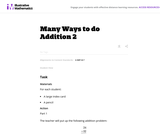Unrestricted Use
CC BY
Rating

This is a task from the Illustrative Mathematics website that is one part of a complete illustration of the standard to which it is aligned. Each task has at least one solution and some commentary that addresses important asects of the task and its potential use. Here are the first few lines of the commentary for this task: Materials For each student: * A large index card * A pencil Action Part 1 The teacher will put up the following addition problem: \begin{align} 24 &\ \...

Subject:
Mathematics
Material Type:
Activity/Lab
Provider:
Illustrative Mathematics
Provider Set:
Illustrative Mathematics
Author:
Illustrative Mathematics
03/25/2014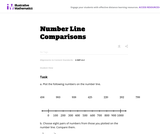Unrestricted Use
CC BY
Rating

This is a task from the Illustrative Mathematics website that is one part of a complete illustration of the standard to which it is aligned. Each task has at least one solution and some commentary that addresses important asects of the task and its potential use. Here are the first few lines of the commentary for this task: Plot the following numbers on the number line. 456 \ \ 983\ \ 938 \ \ 425 \ \ 220 \ \ 202\ \ 799 Choose eight pairs of numbers from those you plotted o...

Subject:
Mathematics
Material Type:
Activity/Lab
Provider:
Illustrative Mathematics
Provider Set:
Illustrative Mathematics
Author:
Illustrative Mathematics
04/04/2014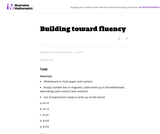Unrestricted Use
CC BY
Rating

This is a task from the Illustrative Mathematics website that is one part of a complete illustration of the standard to which it is aligned. Each task has at least one solution and some commentary that addresses important asects of the task and its potential use. Here are the first few lines of the commentary for this task: Materials * Whiteboard or chart paper and markers * Empty number line or magnetic cubes lined up on the whiteboard, alternating colors every 5 (see sol...

Subject:
Mathematics
Material Type:
Activity/Lab
Provider:
Illustrative Mathematics
Provider Set:
Illustrative Mathematics
Author:
Illustrative Mathematics
06/23/2013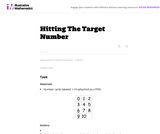Unrestricted Use
CC BY
Rating

This is a task from the Illustrative Mathematics website that is one part of a complete illustration of the standard to which it is aligned. Each task has at least one solution and some commentary that addresses important asects of the task and its potential use. Here are the first few lines of the commentary for this task: Materials * Number cards labeled 1-10 (attached as a PDF) Actions * Begin by playing the game as a whole class to demonstrate the rules and for student...

Subject:
Mathematics
Material Type:
Activity/Lab
Provider:
Illustrative Mathematics
Provider Set:
Illustrative Mathematics
Author:
Illustrative Mathematics